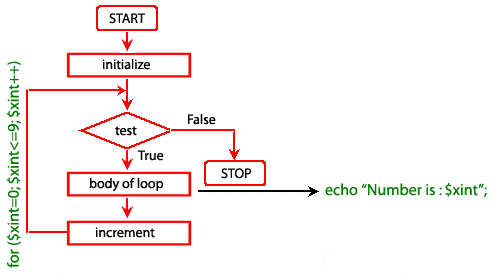# PHP for Loop

## Description

The PHP for loop allows the user to put all the loop-related statements (i.e. INITIALIZER; CONDITION; INCREMENTOR or DECREMENTOR) in one place. The structure is similar to C language.

Syntax

```for ( initialize counter ; test counter ; increment counter)
{
execute the statement;
}
```
• initialize counter : Initialize the loop counter value.
• test counter : Verify the loop counter whether the condition is true.
• increment counter : Increasing the loop counter value.
• execute the statement : Execute php statements.

Alternatively, you can write for loop statement using the following syntax also.

```for (expr1; expr2; expr3):
statement
...
endfor;
```

Pictorial representation of for loopExample:

The following example displays the numbers between 0 to 5.

``````<?php
for (\$xint=0; \$xint<=5; \$xint++)
{
echo "Number is : \$xint <br />";
}
?>``````

Output:

```Number is : 0
Number is : 1
Number is : 2
Number is : 3
Number is : 4
Number is : 5 ```

View the example in the browser

You can execute for in the following ways also.

``````<?php
for (\$xint = 1; ; \$xint++) {
if (\$i >= 5) {
break;
}
echo \$xint;
}
?>``````

``````<?php
\$xint = 1;
for (; ; )
{
if (\$xint >= 5)
{
break;
}
echo \$xint;
\$xint++;
}
?>``````

Example: Counting number of times a word present in a sentence.

``````<?php
\$text="The quick brown Fox jumps over the lazy Dog";
\$words = explode(" ", \$text); // explode function looks for " " and creates an array, where each word is an element of the array
\$now = count(\$words);
\$j = 0;
for(\$i=0; \$i<\$now; \$i++)
{
if (\$words[\$i] == "the" or \$words[\$i] == "The")
{
\$j =  \$j+1;
}
}
echo \$j;
?>``````

Output:

`2`

View the example in the browser

﻿

New Content: Composer: Dependency manager for PHP, R Programming